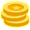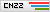# 利用RITA检测beacon通信

|## 前言

RITA 是一个用于检测 C2 通信流量的开源项目，由 GO 语言编写

## tsScore的计算

### tsSkewScore

CS 默认回连的间隔时间为 60 秒，即使加上抖动(jitter)，理论上仍然会呈现出高度对称的分布特征，其数据包大小亦如是

Python 示例代码：

``````# 根据时间戳计算通信间隔
http_df['deltas'] = http_df[timestamp].apply(lambda x: pd.Series(x).diff().dt.seconds.dropna().tolist())
# 以通信间隔为数据集，计算各分位点处数值
http_df['tsLow'] = http_df['deltas'].apply(lambda x: np.percentile(np.array(x), 25))
http_df['tsMid'] = http_df['deltas'].apply(lambda x: np.percentile(np.array(x), 50))
http_df['tsHigh'] = http_df['deltas'].apply(lambda x: np.percentile(np.array(x), 75))
# 根据公式：Bowley Skewness = (Q1 + Q3 – 2Q2) / (Q3 – Q1)，计算分母和分子
http_df['tsBowleyNum'] = http_df['tsLow'] + http_df['tsHigh'] - 2*http_df['tsMid']
http_df['tsBowleyDen'] = http_df['tsHigh'] - http_df['tsLow']
# 如果分母为 0，Q2 = Q1 或 Q2 = Q3，则计算结果不可靠，tsSkew 取 0
http_df['tsSkew'] = http_df[['tsLow','tsMid','tsHigh','tsBowleyNum','tsBowleyDen']].apply(
lambda x: x['tsBowleyNum'] / x['tsBowleyDen'] if x['tsBowleyDen'] != 0 and x['tsMid'] != x['tsLow'] and x['tsMid'] != x['tsHigh'] else 0.0, axis=1
)
``````

``````http_df['tsMadm'] = http_df['deltas'].apply(lambda x: np.median(np.absolute(np.array(x) - np.median(np.array(x)))))
``````

``````http_df['tsMadmScore'] = 1.0 - http_df['tsMadm'] / 30.0
``````

### tsConnCountScore

PS：对于这里的 10 秒，应该还存在优化空间，有研究员指出该值太小可能会影响最终得分从而产生漏报，传送地址

## dsScore的计算

Python 示例代码如下：

``````# 计算数据包大小差值
http_df['size_deltas'] = http_df[data_size].apply(lambda x: pd.Series(x).diff().dropna().tolist())
# 计算 dsSkew
http_df['dsLow'] = http_df['size_deltas'].apply(lambda x: np.percentile(np.array(x), 25))
http_df['dsMid'] = http_df['size_deltas'].apply(lambda x: np.percentile(np.array(x), 50))
http_df['dsHigh'] = http_df['size_deltas'].apply(lambda x: np.percentile(np.array(x), 75))
http_df['dsBowleyNum'] = http_df['dsLow'] + http_df['dsHigh'] - 2*http_df['dsMid']
http_df['dsBowleyDen'] = http_df['dsHigh'] - http_df['dsLow']
http_df['dsSkew'] = http_df[['dsLow','dsMid','dsHigh','dsBowleyNum','dsBowleyDen']].apply(
lambda x: x['dsBowleyNum'] / x['dsBowleyDen'] if x['dsBowleyDen'] != 0 and x['dsMid'] != x['dsLow'] and x['dsMid'] != x['dsHigh'] else 0.0, axis=1
)
# 计算 dsSkewScore
http_df['dsSkewScore'] = 1.0 - abs(http_df['dsSkew'])
http_df['dsMadm'] = http_df['size_deltas'].apply(lambda x: np.median(np.absolute(np.array(x) - np.median(np.array(x)))))
# 计算 dsSmallnessScore
http_df['dsSmallnessScore'] = http_df[data_size].apply(lambda x: 1- (np.argmax(np.bincount(x)) / 65535))
http_df['dsSmallnessScore'] = http_df['dsSmallnessScore'].apply(lambda x: 0 if x < 0 else x)
``````

## 小结

4赞慕长风

## 发表评论### TA的文章

• ##### 针对Office宏病毒的高级检测

2022-02-18 14:30:45

• ##### 利用RITA检测beacon通信

2022-01-29 14:30:41

• ##### 走进Windows中的提权行为

2022-01-18 14:30:09

• ##### 揭秘rundll32中的攻防对抗

2021-12-29 14:30:56

• ##### 基于异常行为检测CobaltStrike

2021-12-14 14:30:23

### 相关文章

• ##### 2023热血再燃|网络安全攻防演练实战公开课来袭！

2023-03-21 17:00:50

• ##### 【OSCP3小时超长直播】「Kali大神」苑房弘带你轻松备考

2023-02-25 12:00:13

• ##### 实战记录之曲线救国

2023-01-11 17:30:42

• ##### 关于bypassuac的探究

2022-10-09 12:00:07

• ##### 弱密码测试工具blaster使用演示

2022-10-08 12:00:34

• ##### 机器渗透与人工渗透的博弈

2022-09-14 13:44:20

• ##### 自动化测试新视角：以SaaS模式检测内网安全

2022-09-01 11:00:20

### 热门推荐

• 投稿须知
• 转载须知
• 官网QQ群8：819797106
• 官网QQ群3：830462644(已满)
• 官网QQ群2：814450983(已满)
• 官网QQ群1：702511263(已满)
Copyright © 北京奇虎科技有限公司 360网络攻防实验室 安全客 All Rights Reserved 京ICP备08010314号-66